One of the first things I learned in SAS was how to use PROC PRINT to display parts of a data set. I usually do not need to see all the data, so my favorite way to use PROC PRINT is to use the OBS= data set option to display the first few rows. For example, I often display the first five rows of a SAS data set as follows:

```proc print data=Sashelp.Class(obs=5); * VAR Weight Height Age; /* optional: the VAR statement specifies variables */ run;```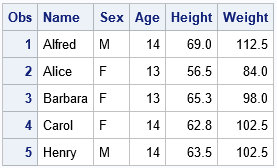By using the OBS= data set option, you can display only a few observations. This enables you to take a quick peek at the values of your data. As shown in the comment, you can optionally use the VAR statement to display only certain columns. (Use the FIRSTOBS= option if you want more control over the rows that are printed.)

### Display the rows of a data table in SAS/IML

In a SAS/IML program, data are either stored in a table or in a matrix. If the data are in a table, you can use the TABLEPRINT subroutine to display the data. The NUMOBS= option enables you to display only a few rows:

```proc iml; TblClass = TableCreateFromDataset("sashelp", "class"); run TablePrint(TblClass) numobs=5;```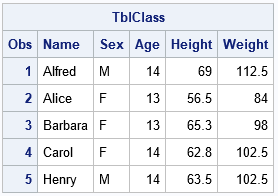The TABLEPRINT subroutine supports many options for printing, including the VAR= option for specifying only certain columns.

### Display the rows of a matrix in SAS/IML

How can you display only a portion of a SAS/IML matrix? I often write statements like this to print only the first few rows:

```/* read numerical data into the X matrix */ use Sashelp.Class; read all var _NUM_ into X[c=varNames]; close; print (X[1:5,]); /* print only rows 1 through 5 */```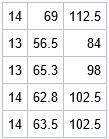This works, but there are a few things that I don't like. My primary complaint is that X[1:5,] is a temporary matrix and therefore has no name. The rows are printed, but there is no header that tells me where the data came from. My second complaint is that the output does not indicate which rows are being displayed. Consequently, sometimes I include information in the label and add row and column headers:

`print (X[1:5,])[rowname=(1:5) colname=varNames label="Top of X"];`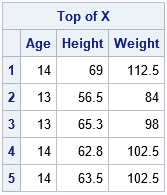The output now includes the information that I want, but that is a LOT of typing, especially if I want to display similar information for other matrices. Even if I use the abbreviated version of the PRINT options (R=, C=, and L=), it is cumbersome to type.

By the way, this PRINT statement demonstrates a new feature of SAS/IML 15.1 (which was released with SAS 9.4M6), which is that the ROWNAME= and COLNAME= options on the PRINT statement support numerical vectors. If you have an earlier version of SAS/IML, you can use rowname=(char(1:5)).

### HEAD: A module to print the top rows of a matrix

There's a saying among computer programmers: if you find yourself writing the same statements again and again, create a function to do it. So, let's write a SAS/IML module to print the top rows of a matrix. Because there is a UNIX command called 'head' that displays the top lines of a file, I will use the same name.

Many years ago, I blogged about how to write a HEAD subroutine, although my emphasis was on how to use default arguments in SAS/IML functions. The following routine is a richer version of the previous function:

```/* Print the first n rows of a matrix. Optionally, display names of columns */ start Head(x, n=5, colname=); m = min(n, nrow(x)); /* make sure n isn't too big */ idx = 1:m; /* the rows to print */ name = parentname("x"); /* name of symbol in calling environment */ if name=" " then name = "Temp"; /* the parent name of a temporary variable is " "*/ labl = "head(" + name + ") rows=" + strip(char(m)); /* construct the label */ if isSkipped(colname) then /* print the top rows */ print (x[idx,])[r=idx label=labl]; else print (x[idx,])[r=idx c=colname label=labl]; finish;   run Head(X) colname=varNames; /* example: call the HEAD module */```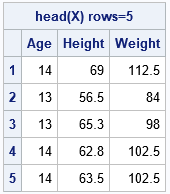The HEAD subroutine uses three features of user-defined modules that you might not know about:

The result is a short way to display the top few rows of a matrix.

### TAIL: A module to print the bottom rows of a matrix

Although I usually want to print the top row of a matrix, it is easy to modify the HEAD module to display the last n rows of a matrix. The following module, called TAIL, is almost identical to the HEAD module.

```/* Print the last n rows of a matrix. Optionally, display names of columns */ start Tail(x, n=5, colname=); m = min(n, nrow(x)); /* make sure n isn't too big */ idx = (nrow(x)-m+1):nrow(x); /* the rows to print */ name = parentname("x"); /* name of symbol in calling environment */ if name=" " then name = "Temp"; /* the parent name of a temporary variable is " "*/ labl = "tail(" + name + ") rows=" + strip(char(m)); /* construct the label */ if isSkipped(colname) then /* print the bottom rows */ print (x[idx,])[r=idx label=labl]; else print (x[idx,])[r=idx c=colname label=labl]; finish;   run Tail(X) colname=varNames;```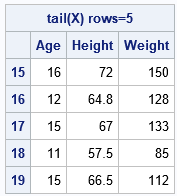### Summary

Most SAS programmers know how to use the OBS= option in PROC PRINT to display only a few rows of a SAS data set. When writing and debugging programs in the SAS/IML matrix language, you might want to print a few rows of a matrix. This article presents the HEAD module, which displays the top rows of a matrix. For completeness, the article also defines the TAIL module, which displays the bottom rows of a matrix. If you find these modules useful, you can incorporate them into your SAS/IML programs.

For more tips and techniques related to SAS/IML modules, see the article "Everything you wanted to know about writing SAS/IML modules."

The post Print the top rows of your SAS data appeared first on The DO Loop.